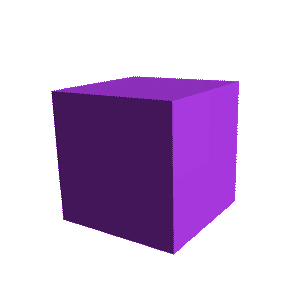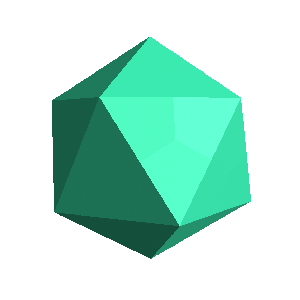Up: The Platonic Solids

# Pictures of the Platonic SolidsThe tetrahedron is the Platonic solid with three triagular faces arranged around every vertex. Plato identified this polyhedron with the shape of fire atoms. The cube is the Platonic solid with three square faces arranged around every vertex. Plato idenfied this polyhedron with the shape of earth atoms.The octahedron is the Platonic solid with four triangular faces arranged around every vertex. Plato idenfied this polyhedron with the shape of air atoms. The icosahedron is the Platonic solid with five triangular faces arranged around every vertex. Plato idenfied this polyhedron with the shape of water atoms.The dodecahedron is the Platonic solid with three pentagonal faces arranged around every vertex. Plato identified this polyhedron with the shape of cosmos atoms.

Go back to the Platonic Solids